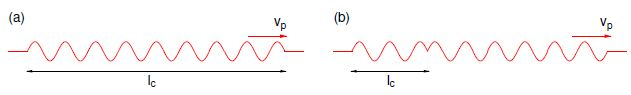Mathematics

# What is Coherence of EM Waves?

The monochromatic plane wave solutions are an idealisation because they are of infinite extent in all directions and are pure sinusoidal waves, i.e. the spread in angular frequencies is Δω = 0. The same is true of monochromatic spherical waves. Such waves are completely coherent Two identical copies of such waves, say each with amplitude E0 and peak intensity I0 = (Ɛ0/2)E02 c, can be made to interfere in a predictable way and show interference fringes alternating between 100% constructive interference with Imax = (Ɛ0/2) (E0 + E0)2 c = 4 I0 and 100% destructive interference with Imin = (Ɛ0/2) (E0 + E0)2 c = 0, such that the visibility of the fringes is

V ≡ (Imax – Imin) / (Imax + Imin) = 1

Real EM waves are only partially coherent, but they may display coherence at a particular location over a limited time called the coherence time to, due to a partially coherent wave train of length equal to the coherence length lc = vpte passing through that location. The width of the wave front across which coherence is maintained is called the coherence width dc. The criterion for determining these paramaters is the time, or distance, over which the phase differs significantly from that of a pure sinusoidal wave. The coherence time, length or width may be used to determine the conditions under which a monochromatic EM plane wave may be a good approximation to the EM field present.

Spectral line emission can be considered quasi-monochromatic, but even then there will be a narrow range of frequencies about the nominal transition frequency. Thus any source of EM waves will in practice produce waves of finite extent and have a spread of frequencies. For example, for an atomic transition the natural line width is Δω ≈ Г where Г is the spontaneous transition rate (Einstein A coefficient for spontaneous emission).

Fourier theory shows that Δ x Δ kx ≈ 1. Given that vp = ω/k, and that for a wave travelling in the x direction kx = k, it follows that Δkx = Δw/vp such that the wave trains associated with emitted photons will have a coherence length

lc = Δx ≈ vp

as illustrated in below Figure. As an example, consider the Sodium D lines which have λ ≈ 590 nm and Г ≈ 6.1 x 107 s-1, for which we find that lc ≈ 5 m or about 8 million wavelengths.Figure: (a) A finite EM wave train due to an atomic transition. (b) A phase shift resulting from a collision during the transition. The coherence length lc, is the smallest coherent part of the wave train.

In practice, the coherence time will be shorter and the observed width of a spectral line broader. If, for example, during the transition the atom collides with another atom then a phase shift is introduced, and the coherence length will be reduced accordingly (Fig. b). This effect becomes more important with increasing density and temperature and is referred to as pressure broadening. Also, because of thermal motion, the frequency of a photon emitted by an individual atom will be doppler shifted by an amount depending on the atom’s component of velocity toward the observer. This Doppler broadening produces a gaussian line shape with line-width proportional to √T’.